A pan-cancer analysis relating microsatellite instability to expression patterns across 33 tumors

VJ Carey [email protected]

This notebook uses information created in the pancan_tx2 workspace with resources registered at dockstore.org in the msireg1 workflow. Specifically, the WDL registered at msireg1 uses Bioconductor's curatedTCGAData and BiocOncoTK packages to collect all RNASeq2GeneNorm measures for 21 genes on each of 9533 tumors from 33 TCGA tumor types, binding MSIsensor scores to all records as continuous measures of microsatellite instability.

## Basic data processing¶

In :
fn = "gs://bioc_derived_data/msi_ex_33t_21g.rds"


### Ingestion and description of workflow output¶

In :
system(paste("gsutil cp ", fn, " ."), intern=TRUE)

In :
library(dplyr, quietly=TRUE)
glimpse(newdf)
length(unique(newdf$patient_barcode))  Attaching package: ‘dplyr’ The following objects are masked from ‘package:stats’: filter, lag The following objects are masked from ‘package:base’: intersect, setdiff, setequal, union  Observations: 200,193 Variables: 8$ X               <int> 1, 2, 3, 4, 5, 6, 7, 8, 9, 10, 11, 12, 13, 14, 15, 16…
$patient_barcode <chr> "TCGA-OR-A5J1", "TCGA-OR-A5J2", "TCGA-OR-A5J3", "TCGA…$ acronym         <chr> "ACC", "ACC", "ACC", "ACC", "ACC", "ACC", "ACC", "ACC…
$symbol <chr> "FOXM1", "FOXM1", "FOXM1", "FOXM1", "FOXM1", "FOXM1",…$ alias           <chr> "FOXM1", "FOXM1", "FOXM1", "FOXM1", "FOXM1", "FOXM1",…
$log2ex <dbl> 6.252206, 9.708816, 7.809840, 10.016878, 4.811296, 11…$ msival          <dbl> 0.00, 1.57, 1.82, 5.38, 0.00, 0.79, 0.64, 0.90, 0.72,…
$msicode <chr> "<4", "<4", "<4", ">=4", "<4", "<4", "<4", "<4", "<4"…  9533 ### Filtering to primary tumors; addition of plate identifiers¶ In the following chunk, we obtain sample barcodes, from which we can derive contribution type and plate identifiers, merge these with the workflow output, and filter the workflow output to primary tumor contributions. In : #source("plate_run.R", echo=TRUE) system("gsutil cp gs://bioc_derived_data/all_plates.rda .", intern=TRUE) load("all_plates.rda") allp = do.call(rbind,all_plates) mx = merge(newdf, allp) # has multiple contributions from some patients stype=substr(mx$sample_barcode,14,15)
mx$stype = stype dta = which(table(mx$patient_barcode)==42)
dups = names(dta)
dd = mx[mx$patient_barcode%in%dups,] # table(dd$patient_barcode, dd$stype) -- shows they all have "01" nondup = setdiff(mx$patient_barcode, dups)
mx2 = mx %>% filter(patient_barcode %in% nondup|stype == "01")

singplates = filter(mx2, acronym %in% c("ACC", "CHOL", "UCS", "UVM"))
multplates = filter(mx2, !(acronym %in% c("ACC", "CHOL", "UCS", "UVM")))


### Basic visualizations¶

#### Surveys of sample numbers and MSIsensor scores per tumor type¶

In :
library(ggplot2, quietly=TRUE)
options(repr.plot.width=4.8)
one_rec_per_sample = mx2 %>% filter(symbol=="FOXM1")
ntum = ggplot(one_rec_per_sample, aes(factor(acronym))) + geom_bar() +
coord_flip() + xlab("TCGA tumor type") + ggtitle("Number of samples per tumor type")

In :
msidists = ggplot(one_rec_per_sample, aes(y=log10(msival+1), x=factor(acronym))) +
geom_boxplot() + coord_flip() + ggtitle("Distributions of MSIsensor scores")


The figure to the right below is very similar to Figure 5B of Bailey

In :
library(ggpubr)
options(repr.plot.width=14,repr.plot.height=7)
ggarrange(ntum,msidists,nrow=1)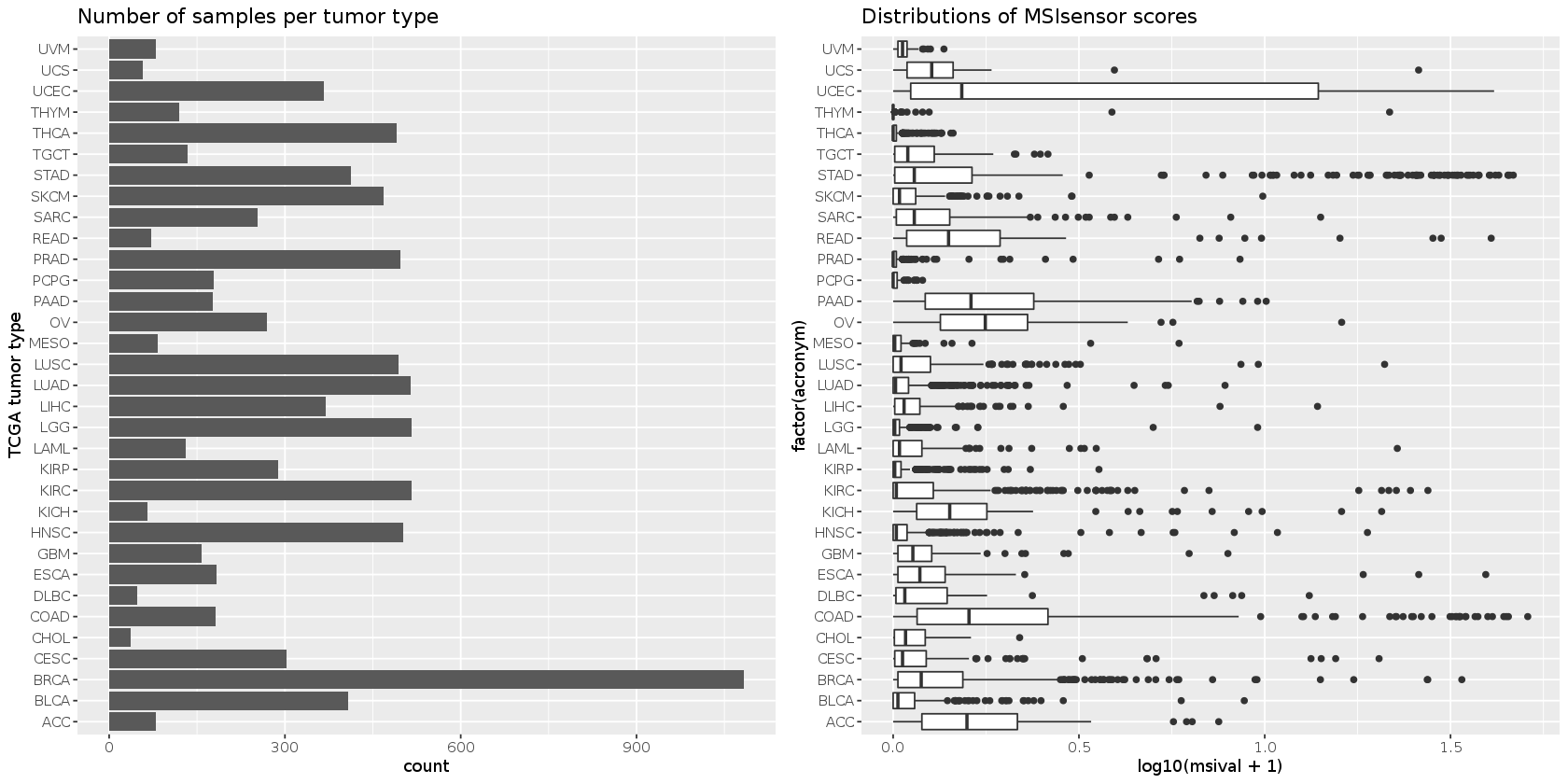#### Bimodality in MSI distributions: two examples¶

A very weak signal of bimodality of MSIsensor scores is seen in KIRC (Renal Clear Cell Carcinoma); this is more pronounced in stomach cancer.

In :
options(repr.plot.width=14)
kirh = ggplot(one_rec_per_sample %>%
filter(acronym=="KIRC"), aes(x=log10(msival+1))) + geom_histogram() + ggtitle("MSI in KIRC")
stah = ggplot(one_rec_per_sample %>%
ggarrange(kirh,stah,nrow=1)

stat_bin() using bins = 30. Pick better value with binwidth.

stat_bin() using bins = 30. Pick better value with binwidth.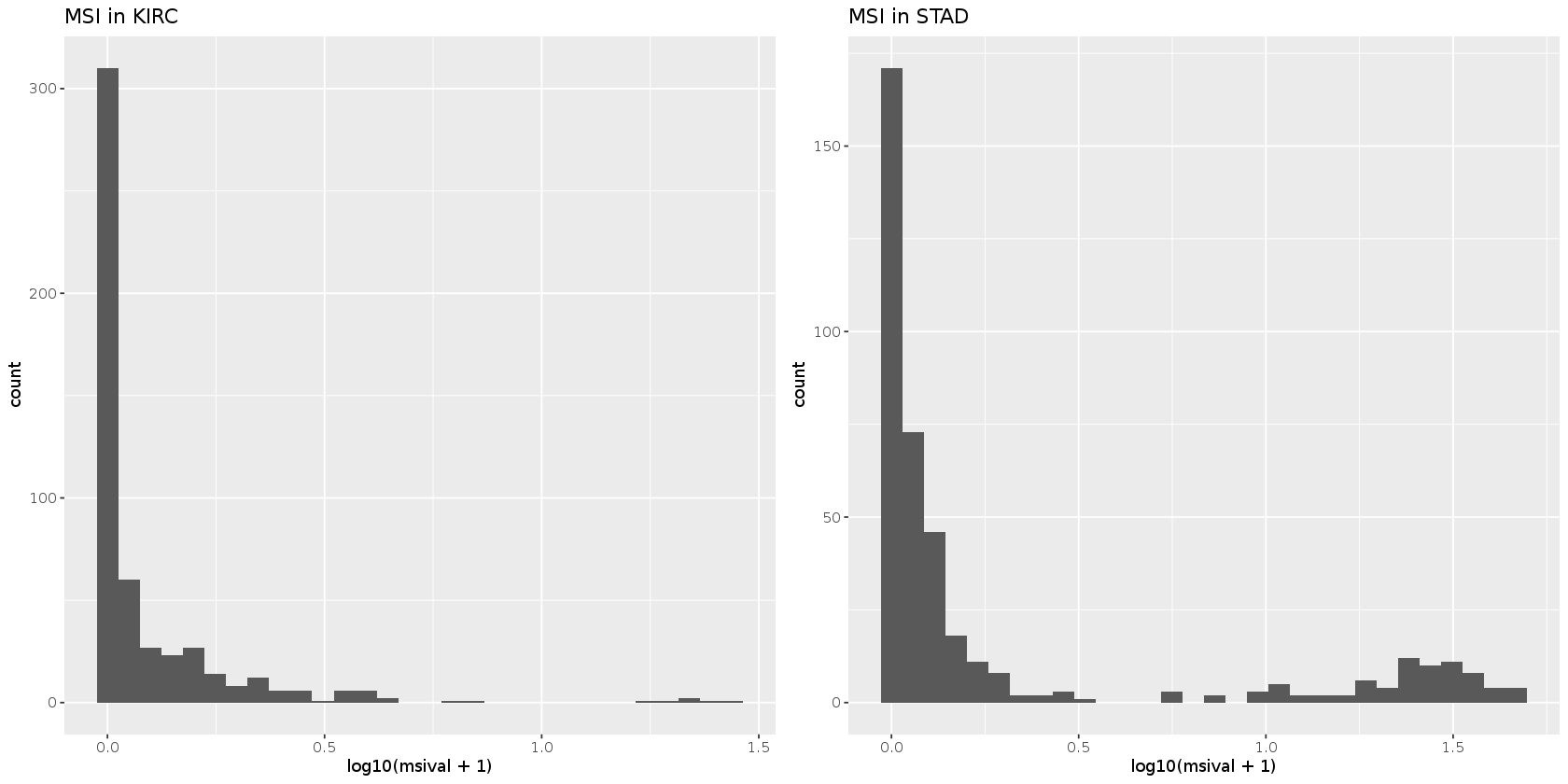There is some controversy over the role of MSI in hematologic malignancies (e.g., Walker et al., 2017). Numbers are smaller in two examples checked here.

In :
options(repr.plot.width=14)
kirh = ggplot(one_rec_per_sample %>%
filter(acronym=="DLBC"), aes(x=log10(msival+1))) + geom_histogram() + ggtitle("MSI in DLBC")
stah = ggplot(one_rec_per_sample %>%
filter(acronym=="LAML"), aes(x=log10(msival+1))) + geom_histogram() + ggtitle("MSI in LAML")
ggarrange(kirh,stah,nrow=1)

stat_bin() using bins = 30. Pick better value with binwidth.

stat_bin() using bins = 30. Pick better value with binwidth.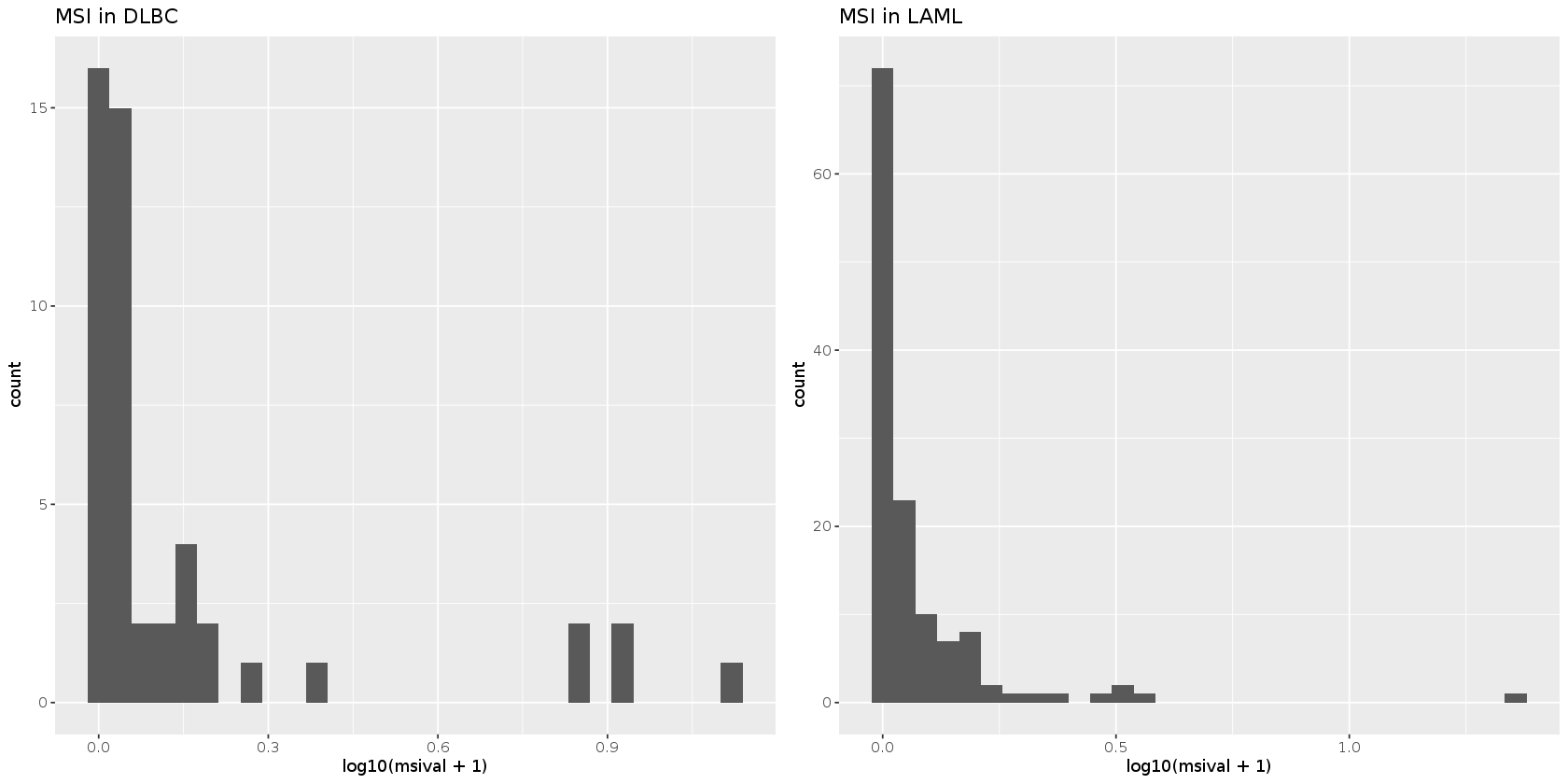Bailey et al. 2018 remark that MSIsensor scores less than 4 do not reliably discriminate MSI from MSS. We sketch the tumor-specific densities below, marking with a vertical line the threshold at MSIsensor score 4.

In :
library(scales)
vjctrans = function (p=1)
{
trans <- function(x) {
log10(x+p)
}
inv <- function(x) {
(10^(x))-p
}
trans_new("vjc", trans, inv)
}

In :
ggplot(one_rec_per_sample, aes(x=msival, colour=acronym)) +
geom_density(bw=.1) + ggtitle("MSIsensor scores") + scale_x_continuous(trans=vjctrans()) + geom_vline(xintercept=4)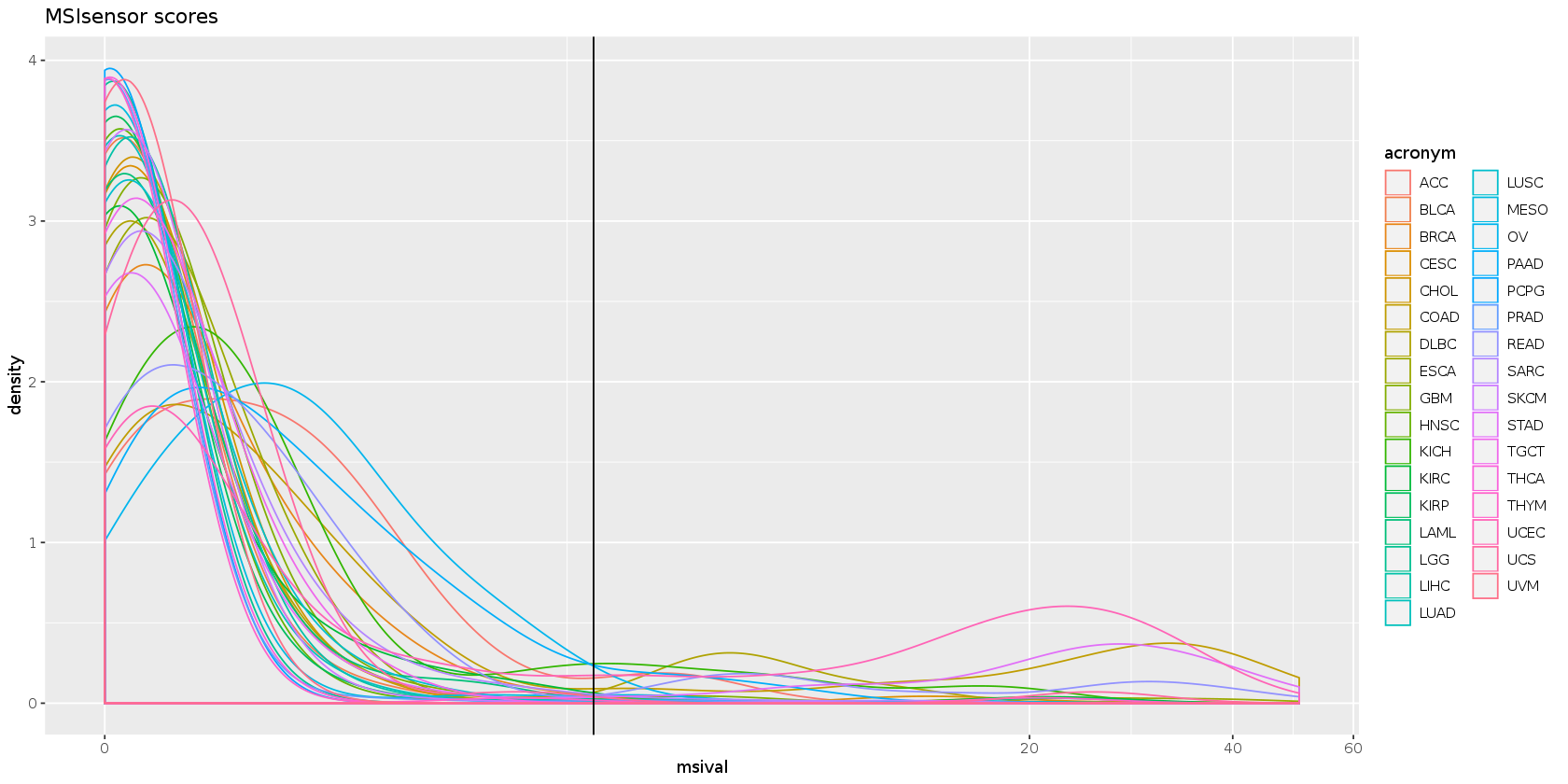## Basic associations between MSI and expression¶

### Overview¶

We set up the basic code for visualizing stratified and regressive evaluation of association between MSI and expression of selected genes within TCGA tumor types.

In :
options(repr.plot.width=14,repr.plot.height=7)
ggbox = function(tumor, gene, titlepref="") ggplot(newdf %>% filter(acronym==tumor&symbol==gene), aes(y=log2ex, x=msicode)) +
geom_boxplot() + ggtitle(paste0(titlepref,tumor)) + ylab(gene) + xlab("MSIsensor category")
ggscat = function(tumor, gene, titlepref="", intrans=vjctrans(p=1),inmeth="auto", topmsi=Inf) ggplot(newdf %>% filter(acronym==tumor&symbol==gene&msival<topmsi), aes(y=log2ex, x=msival)) +
geom_hex() + ggtitle(paste0(titlepref,tumor)) + ylab(gene) + scale_x_continuous(trans=intrans) + geom_smooth(method=inmeth) +
xlab("MSIsensor score")
co = ggbox("COAD", "CD8A", titlepref="a) ")
st = ggbox("STAD", "CD8A", titlepref="b) ")
uc = ggbox("UCEC", "CD8A", titlepref="c) ")
tg = ggscat("TGCT", "CD8A", titlepref="d) ",inmeth=MASS::rlm)
ggarrange(co,st,uc,tg,nrow=2,ncol=2)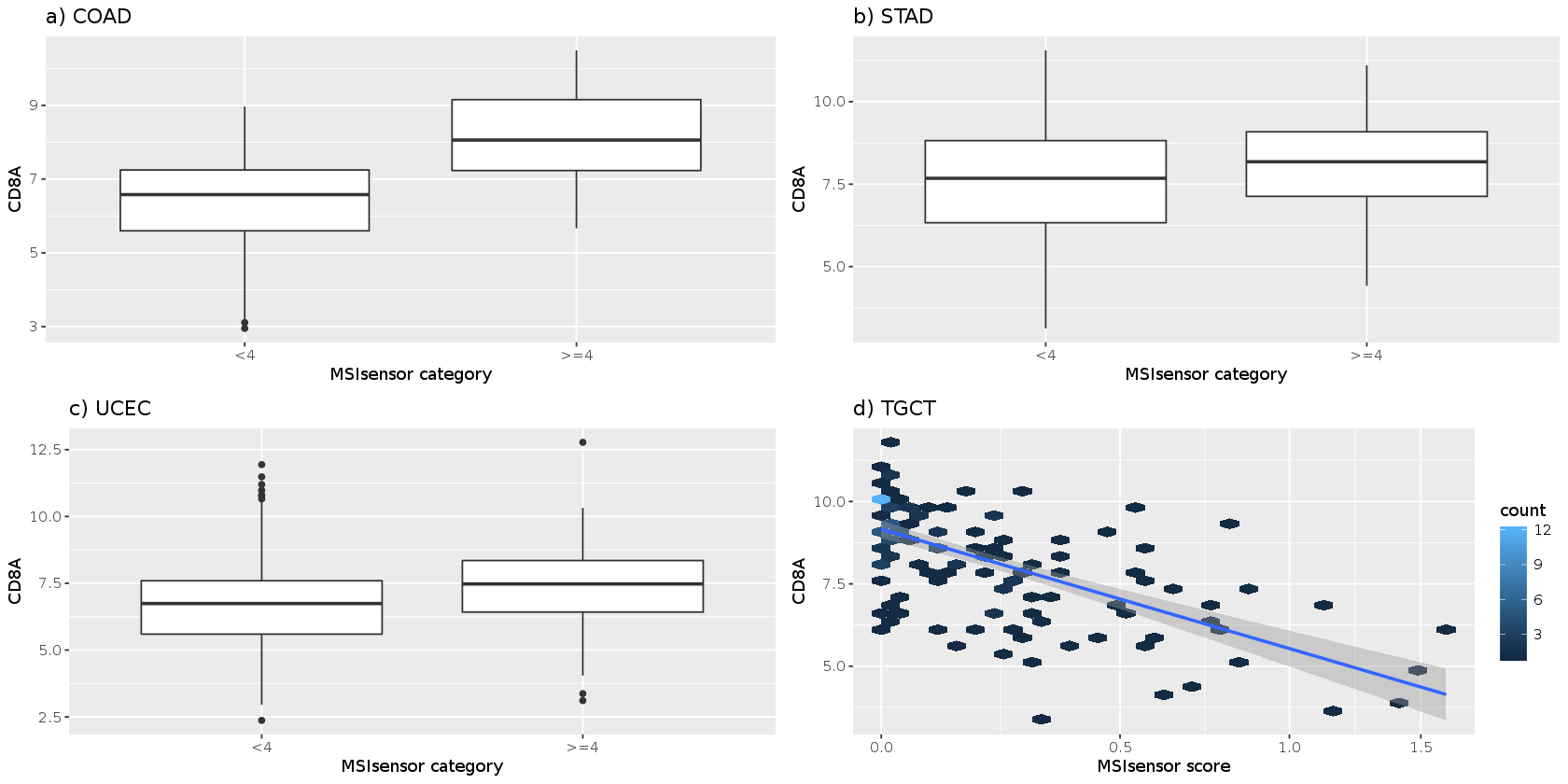We define a function that gives a pair of plots for a given tumor-gene combination, allowing for different transformations, regression displays (parameter inmeth drives the geom_smooth method), and xlims (topmsi parameter defines the upper bound for xlim interval).

In :
demo = function(tum="COAD", gene="TYMS", intrans=vjctrans(p=1), inmeth="auto", topmsi=Inf) {
g1 = ggbox(tum,gene)
g2 = ggscat(tum,gene, intrans=intrans, inmeth=inmeth, topmsi=topmsi)#+geom_vline(xintercept=4)
#ggarrange(g1,g2,nrow=1)
list(g1,g2)
}
options(repr.plot.width=14,repr.plot.height=6)
ggarrange(d1[],d1[],nrow=1)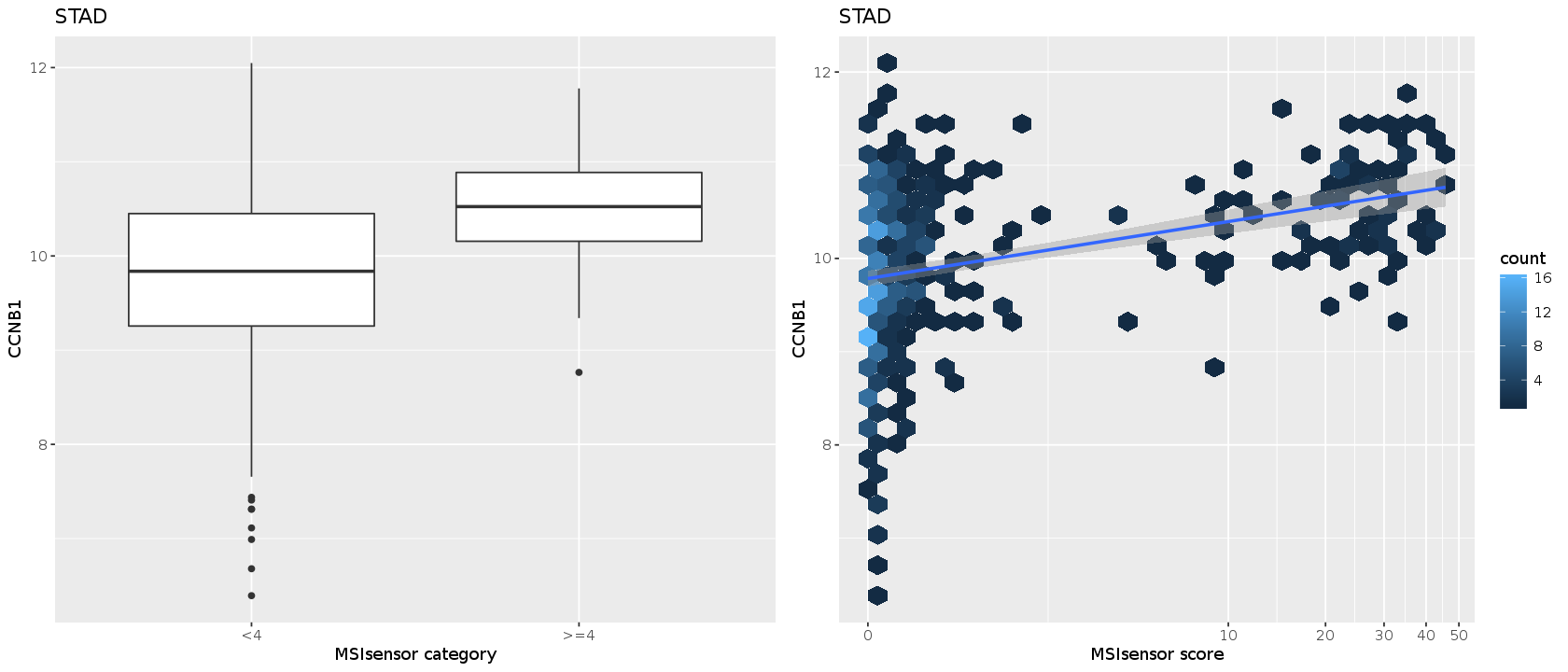### Two-sample tests¶

We now prepare to carry out t tests for association between expression and MSIsensor value, thresholded at 4. The data are coded so that a negative t statistic implies greater mean expression for tumors with MSIsensor score above 4.

In :
tum = unique(newdf$acronym) gn = unique(newdf$alias)
tg = expand.grid(tumor=tum, gene=gn)
i=1
with(newdf %>% filter(acronym==tg$tumor[i]&symbol==tg$gene[i]), t.test(log2ex~msicode))
class(newdf$msicode)   Welch Two Sample t-test data: log2ex by msicode t = -1.7067, df = 3.7588, p-value = 0.1677 alternative hypothesis: true difference in means is not equal to 0 95 percent confidence interval: -2.6497160 0.6640509 sample estimates: mean in group <4 mean in group >=4 8.506112 9.498945  'character' In this chunk we collect t statistics for all gene-tumor pairs. For tumors with no MSIsensor score greater than 4, NAs are returned. In : t1 = function(i) with(newdf %>% filter(acronym==tg$tumor[i]&symbol==tg$gene[i]), { tt = t.test(log2ex~msicode) tstat = tt$statistic
p.val = tt$p.value c(tstat=tstat, pval=p.val) }) allp = sapply(1:nrow(tg), function(x) { ans = try(t1(x),silent=TRUE); if (inherits(ans, "try-error")) return(c(tstat=NA,pval=NA)) ans})  We transform the collection of p-values to Benjamini-Hochberg FDR. In : t.tests = cbind(tg,raw.p.t=allp["pval",],tstat=allp["tstat.t",]) library(multtest) adjp = mt.rawp2adjp(allp["pval",], proc="BH")  Loading required package: BiocGenerics Loading required package: parallel Attaching package: ‘BiocGenerics’ The following objects are masked from ‘package:parallel’: clusterApply, clusterApplyLB, clusterCall, clusterEvalQ, clusterExport, clusterMap, parApply, parCapply, parLapply, parLapplyLB, parRapply, parSapply, parSapplyLB The following objects are masked from ‘package:dplyr’: combine, intersect, setdiff, union The following objects are masked from ‘package:stats’: IQR, mad, sd, var, xtabs The following objects are masked from ‘package:base’: anyDuplicated, append, as.data.frame, basename, cbind, colMeans, colnames, colSums, dirname, do.call, duplicated, eval, evalq, Filter, Find, get, grep, grepl, intersect, is.unsorted, lapply, lengths, Map, mapply, match, mget, order, paste, pmax, pmax.int, pmin, pmin.int, Position, rank, rbind, Reduce, rowMeans, rownames, rowSums, sapply, setdiff, sort, table, tapply, union, unique, unsplit, which, which.max, which.min Loading required package: Biobase Welcome to Bioconductor Vignettes contain introductory material; view with 'browseVignettes()'. To cite Bioconductor, see 'citation("Biobase")', and for packages 'citation("pkgname")'.  In : np = adjp$adjp[order(adjp$index),] np = cbind(t.tests,np) sig.t = np[which(np[,"BH"]<0.05),] sig.t$tumor = as.character(sig.t$tumor) sig.t$gene = as.character(sig.t$gene) dim(sig.t) head(sig.t %>% arrange(BH))  1. 40 2. 6 A data.frame: 6 × 6 tumorgeneraw.p.ttstatrawpBH <chr><chr><dbl><dbl><dbl><dbl> STADTYMS 4.509690e-27-12.7397554.509690e-273.125215e-24 COADTYMS 1.195560e-23-13.5123751.195560e-234.142616e-21 BLCACEP551.878986e-22-10.3554771.878986e-224.340457e-20 STADCCNB12.616038e-17 -9.3372202.616038e-174.532286e-15 STADCEP557.402448e-14 -8.1491237.402448e-141.025979e-11 BLCASATB13.007088e-13 34.0177433.007088e-133.473186e-11 We'll present the results as a bipartite graph. We'll use orange for genes regarded as prognostically unfavorable by Gentles et al. 2015, and blue for the collection of favorable genes along with CD8A. Edges linking tumors with genes for which MSIsensor score is significantly positively associated (t-test based FDR < 0.05) with expression are colored blue; edges corresponding to negative associations are colored orange. In : library(graph) g = new("graphNEL", edgemode="directed", nodes = unique(c(sig.t$tumor,sig.t$gene))) for (i in 1:nrow(sig.t)) g = addEdge(to=sig.t$gene[i], from=sig.t$tumor[i], g)  In : graph::graph.par(list(nodes=list(shape="rectangle", cex=.65, lwd=0))) library(Rgraphviz) g  Loading required package: grid  A graphNEL graph with directed edges Number of Nodes = 29 Number of Edges = 40  In : adv = c("FOXM1", "BIRC5", "TOP2A", "TPX2", "NME1", "CCNB1", "CEP55", "TYMS", "CENPF", "CDKN3") fav = c("KLRB1", "ITM2B", "CBX7", "CD2", "CREBL2", "SATB1", "NR3C1", "TMEM66", "KLRK1", "FUCA1", "CD8A")  Decorating the graph for rendering with Rgraphviz takes a bit of work. We use nodeRenderInfo to color gene names with respect to the Gentles classification, leaving tumor names uncolored. Use of edgeRenderInfo requires construction of edge-element names with the form [tumor]~[gene]. In : #plot(g) gnel = Rgraphviz::layoutGraph(g) nodeRenderInfo(gnel)$fill[] = ifelse(nodes(g) %in% fav, "lightblue",
ifelse(nodes(g) %in% gn, "orange", "transparent"))
enm = paste0(sig.t$tumor,"~",sig.t$gene)
tst = sig.t$tstat edgeRenderInfo(gnel)$col[enm][] = ifelse(tst<0,"orange", "blue") # see note about directionality above
options(repr.plot.width=16,repr.plot.height=7)
Rgraphviz::renderGraph(gnel)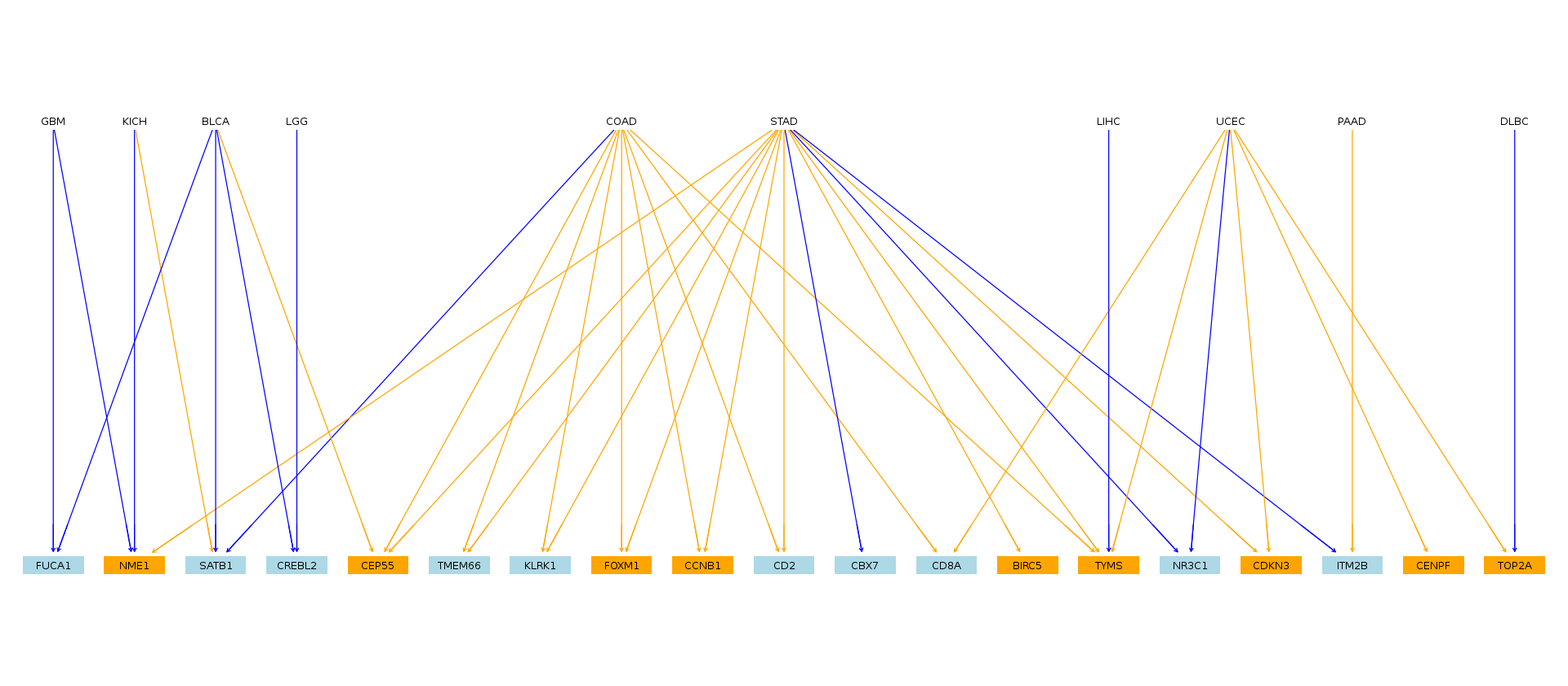To demonstrate how to read the graph, we examine four tumors and the qualitatively different relationships observed between MSI and gene expression. For GBM and KICH, higher MSI is associated with decreased NME1 expression, as indicated by the edges color blue. For STAD and and COAD, higher MSI is associated with increased FOXM1 expression, and the associated edges are orange.

In :
d1 = demo("GBM", "NME1",inmeth=MASS::rlm)
d2 = demo("KICH", "NME1", inmeth=MASS::rlm)
options(repr.plot.width=14,repr.plot.height=6)
ggarrange(d1[],d2[],nrow=1)
ggarrange(d3[],d4[],nrow=1)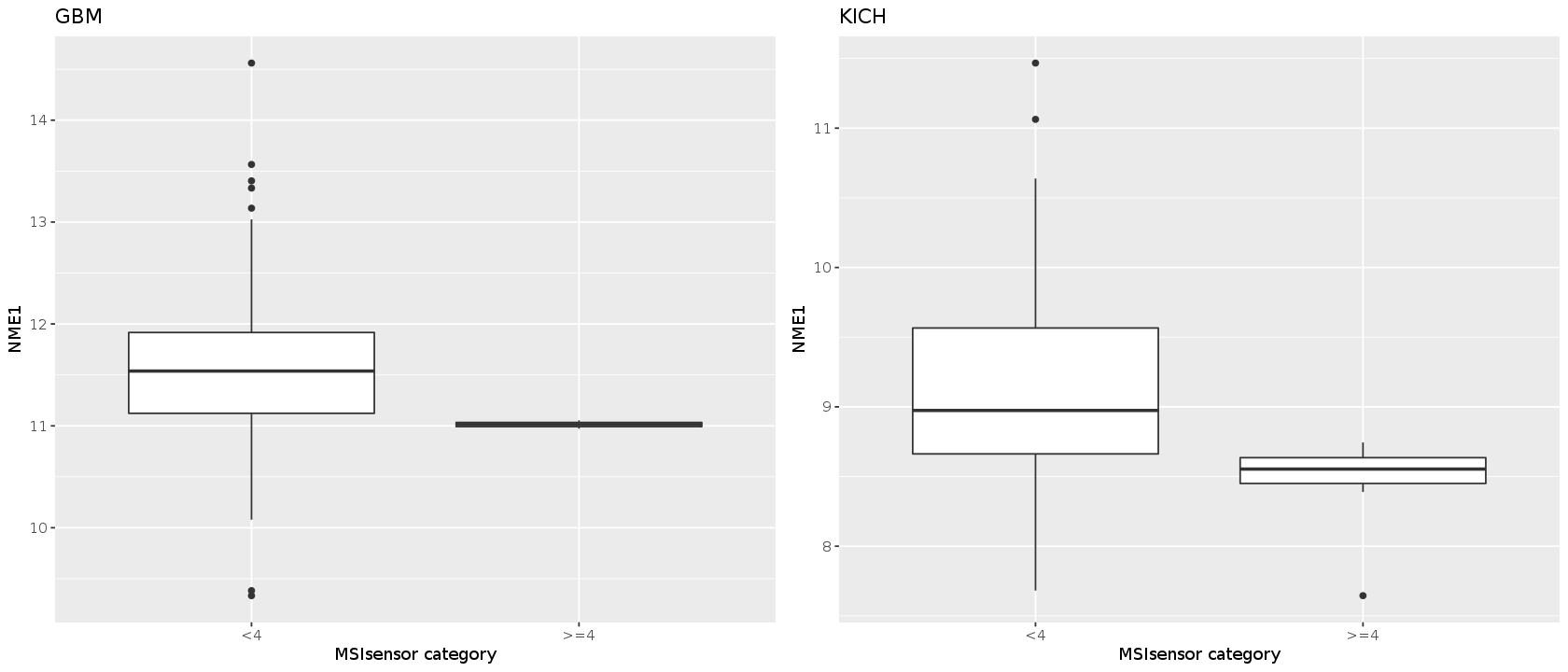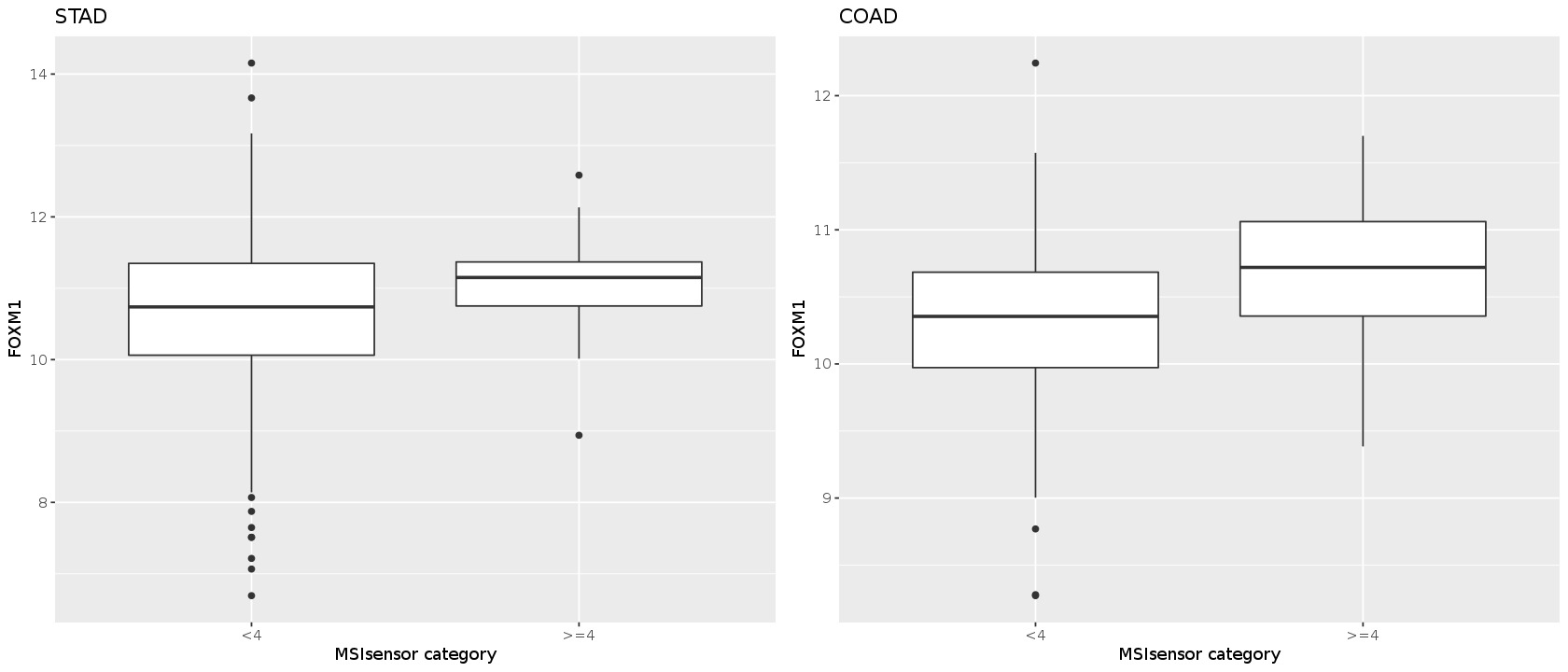### Plate-adjusted linear regression per tumor type¶

In :
library(tidyr)
library(broom)
mods_sing = singplates %>% group_by(acronym,alias) %>%
do(model=lm(log2ex~log(msival+1), data=.))
tmods_sing = tidy(mods_sing, model)
stats_sing = tmods_sing %>% filter(term=="log(msival + 1)")
r2_sing = sapply(mods_sing$model, function(x)summary(x)$adj.r.squared)
stats_sing$r2 = r2_sing mods_mult = multplates %>% group_by(acronym,alias) %>% do(model=lm(log2ex~log(msival+1)+factor(plate), data=.)) tmods_mult = tidy(mods_mult, model) stats_mult = tmods_mult %>% filter(term=="log(msival + 1)") r2_mult = sapply(mods_mult$model, function(x)summary(x)$adj.r.squared) stats_mult$r2 = r2_mult

Attaching package: ‘tidyr’

The following object is masked from ‘package:magrittr’:

extract


In :
mods_unadj = mx2 %>% group_by(acronym,alias) %>%
do(model=lm(log2ex~log(msival+1), data=.))
stats_un = tmods_un %>% filter(term=="log(msival + 1)")
r2_un = sapply(mods_unadj$model, function(x)summary(x)$adj.r.squared)
stats_un$r2 = r2_un  In : allstats = rbind(stats_sing,stats_mult)  In : head(allstats)  A grouped_df: 6 × 8 acronymaliastermestimatestd.errorstatisticp.valuer2 <chr><chr><chr><dbl><dbl><dbl><dbl><dbl> ACCBIRC5log(msival + 1) 0.63286910.5230877 1.2098720.2300293960.005910875 ACCCBX7 log(msival + 1) 0.35687610.2661522 1.3408730.1839041230.010126396 ACCCCNB1log(msival + 1) 0.91654390.2882344 3.1798560.0021234320.104595652 ACCCD2 log(msival + 1)-1.26045210.5843383-2.1570590.0341207280.044736972 ACCCD8A log(msival + 1)-0.88758340.5195090-1.7085050.0915711600.024011661 ACCCDKN3log(msival + 1) 1.11424560.4153297 2.6827980.0089317760.073605659 In : library(multtest) mt = mt.rawp2adjp(allstats$p.value, proc="BH")
myd = mt$adjp[order(mt$index),]
colnames(myd) = c("rawp", "BH")
allst = data.frame(allstats, myd)

A data.frame: 6 × 10
acronymaliastermestimatestd.errorstatisticp.valuer2rawpBH
<chr><chr><chr><dbl><dbl><dbl><dbl><dbl><dbl><dbl>
ACCBIRC5log(msival + 1) 0.63286910.5230877 1.2098720.2300293960.0059108750.2300293960.364783458
ACCCBX7 log(msival + 1) 0.35687610.2661522 1.3408730.1839041230.0101263960.1839041230.308701018
ACCCCNB1log(msival + 1) 0.91654390.2882344 3.1798560.0021234320.1045956520.0021234320.008361015
ACCCD2 log(msival + 1)-1.26045210.5843383-2.1570590.0341207280.0447369720.0341207280.081487305
ACCCD8A log(msival + 1)-0.88758340.5195090-1.7085050.0915711600.0240116610.0915711600.181310898
ACCCDKN3log(msival + 1) 1.11424560.4153297 2.6827980.0089317760.0736056590.0089317760.027881625
In :
sigslopes = allst %>% filter(BH<0.05)
dim(sigslopes)

1. 246
2. 10
A data.frame: 6 × 10
acronymaliastermestimatestd.errorstatisticp.valuer2rawpBH
<chr><chr><chr><dbl><dbl><dbl><dbl><dbl><dbl><dbl>
ACCCCNB1log(msival + 1)0.91654390.28823443.1798560.0021234320.104595650.0021234320.008361015
ACCCDKN3log(msival + 1)1.11424560.41532972.6827980.0089317760.073605660.0089317760.027881625
ACCCENPFlog(msival + 1)1.09008980.39629562.7506990.0074094090.077647250.0074094090.024805413
ACCFOXM1log(msival + 1)1.28165930.37906343.3811210.0011365260.117966160.0011365260.005081372
ACCTOP2Alog(msival + 1)1.30275650.41248573.1583070.0022671520.103189580.0022671520.008728534
ACCTPX2 log(msival + 1)0.92974690.33162332.8036240.0063916560.080842330.0063916560.021819792
In :
slg = new("graphNEL", edgemode="directed",
nodes = unique(c(sigslopes$acronym,sigslopes$alias)))
for (i in 1:nrow(sigslopes)) slg = addEdge(to=sigslopes$alias[i], from=sigslopes$acronym[i], slg)
slg

A graphNEL graph with directed edges
Number of Nodes = 49
Number of Edges = 246 

For the regression tests, a positive slope relating MSIsensor score to

In :
gnel = Rgraphviz::layoutGraph(slg)
nodeRenderInfo(gnel)$fill[] = ifelse(nodes(slg) %in% fav, "lightblue", ifelse(nodes(slg) %in% gn, "orange", "transparent")) enm = paste0(sigslopes$acronym,"~",sigslopes$alias) tst = sigslopes$statistic
edgeRenderInfo(gnel)\$col[enm][] = ifelse(tst>0,"orange", "blue") # see note about directionality above
options(repr.plot.width=12,repr.plot.height=6)
Rgraphviz::renderGraph(gnel)